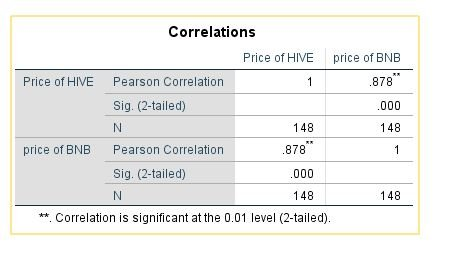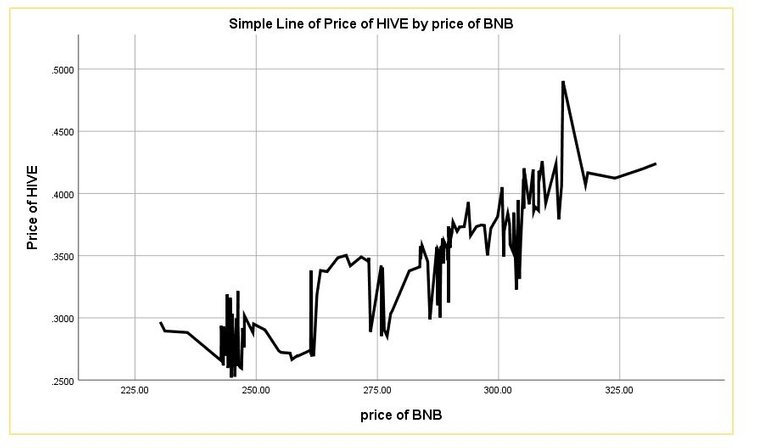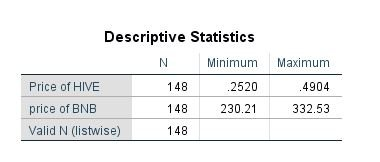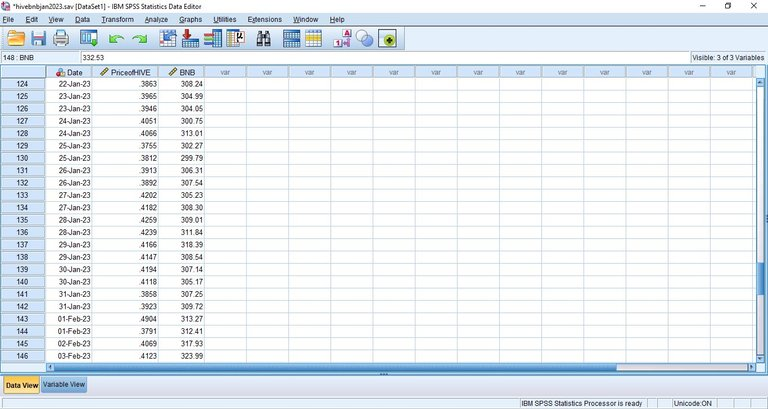# Visualizing the correlation between HIVE and BNBThis moment is a good time for HIVE as analysis presents there is a correlation with BNB too. In this analysis, I will present the level of relationship between HIVE and BNB. The data was sampled from coingecko.com within the time frame of December, 2022 to 3rd of February, 2023.

## Correlation result

Analysis of correlation determines the relationship between HIVE and BNB in the crypto market as regards if there is a pump in the price of BNB, to what extent will it affect the price of HIVE (positively or negatively).

This made a statistician named Karl Pearson, summarized the kinds of correlation between two or more variables to be positive, negative or zero.

A relationship is said to be positive if there is an increase in the value of one will lead to an increase in the value of other and if there is a decrease, same thing will be reflected in the other variable. The value of a positive correlation tends from 0 to +1 on a number scale.

A negative relationship indicates that as the price of one variable increases the other decreases and vice versa. The value of a negative correlation extends from 0 to -1 axis on a number scale.

A zero relationship indicates that no relationship exist at all between both variables. It has a value of 0 on the number scale.

## A case study is between HIVE and BNBConsidering the table, it indicates that with a Pearson correlation coefficient of 0.878 shows a high positive correlation although it’s not perfect. But it can be concluded that as the price of BNB pumps, the price of HIVE also pumps as well at closer range in rate.

Details of this can be discovered from line graph HIVE by BNBThis graph plots the price of BNB on the X axis whilst that of HIVE on the Y axis. You can also visualize that when the price of BNB is on the increase the price of HIVE is likely to also increase although not in every scenario but majority carries a positive correlation between both.

## Maximum and minimum price of both assets

The maximum and minimum price of BNB and HIVE is presented in this section in a table.During the period of December 1 – February, 2023, the highest price of HIVE has been \$0.4904. It is nearly equal to half a dollar, if everything remains fine in the crypto market, it might estimate a dollar. This could be a good season for holders. This price was discovered in 2023.

For BNB the maximum price of BNB was \$332.53, this is a good indication that it may likely estimate to \$350 dollar in the first quarter of the year.
Other details of the minimum prices of both asset is presented on the table.
Statistics such as mean wasn’t included due to the fact that no information can be deducted from it as price isn’t stable.

## Data

The logic behind deriving this information is from the data presented on the spreadsheet.## Conclusively

The relationship between HIVE and BNB was considered in this article, However there seems to be a positive correlation between both assets as at the time the data were sampled.

Posted Using LeoFinance Beta

0
0
0.000